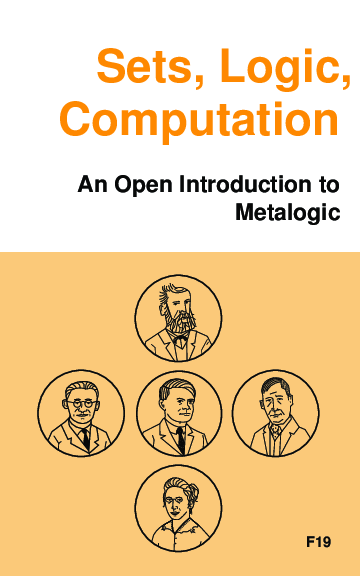# Sets, Logic, Computation. An Open Introduction to Metalogic

Sets, Logic, Computation is an introductory textbook on metalogic. It covers naive set theory, first-order logic, sequent calculus and natural deduction, the completeness, compactness, and Löwenheim-Skolem theorems, Turing machines, and the undecidability of the halting problem and of first-order logic. The audience is undergraduate students with some background in formal logic, e.g., what is covered by forall x.